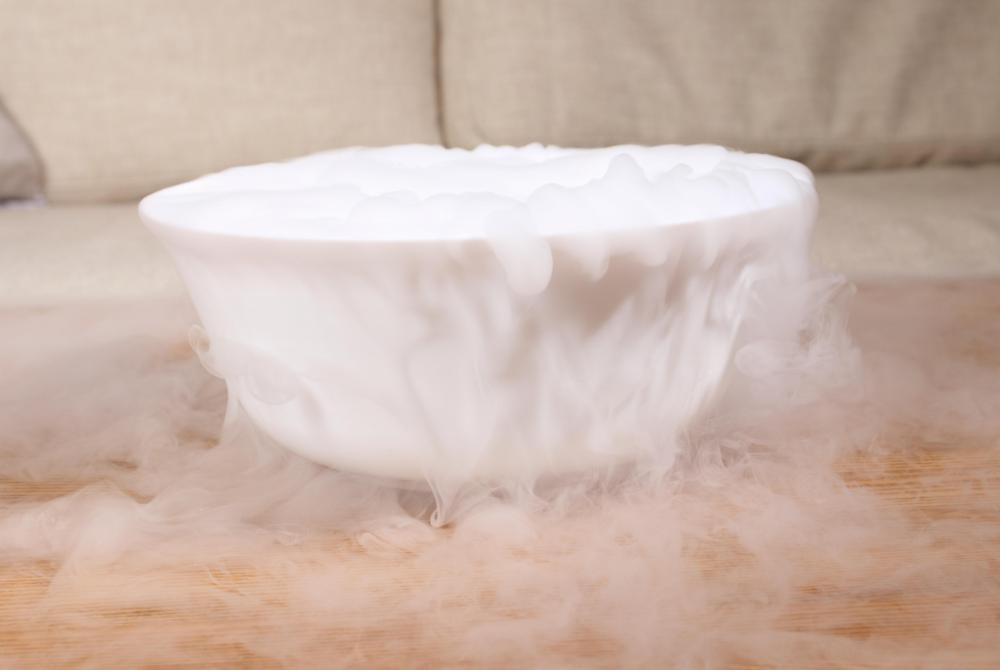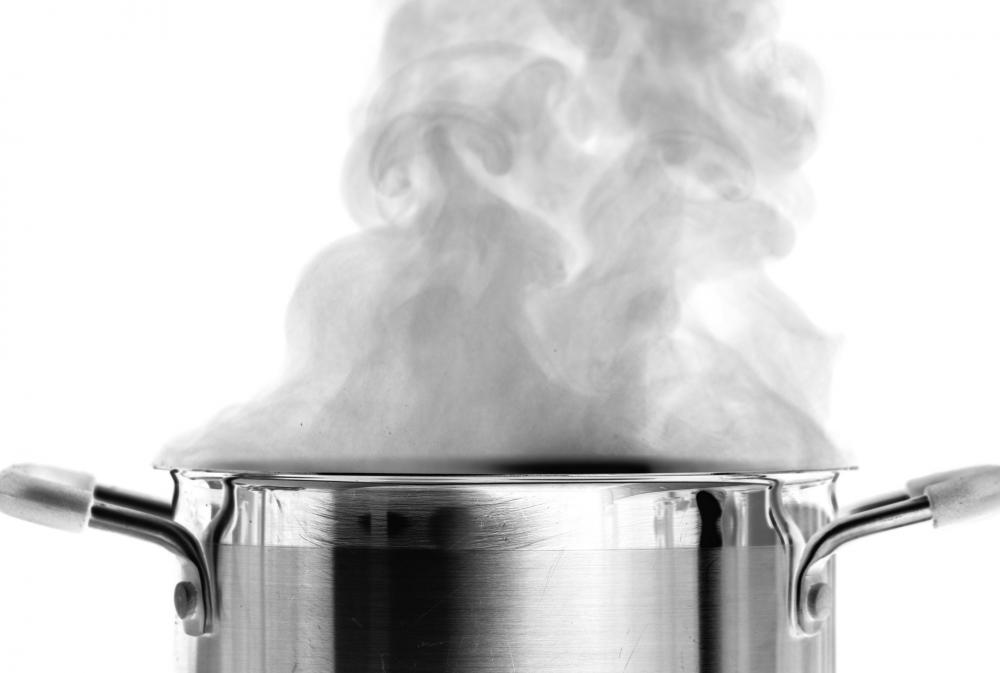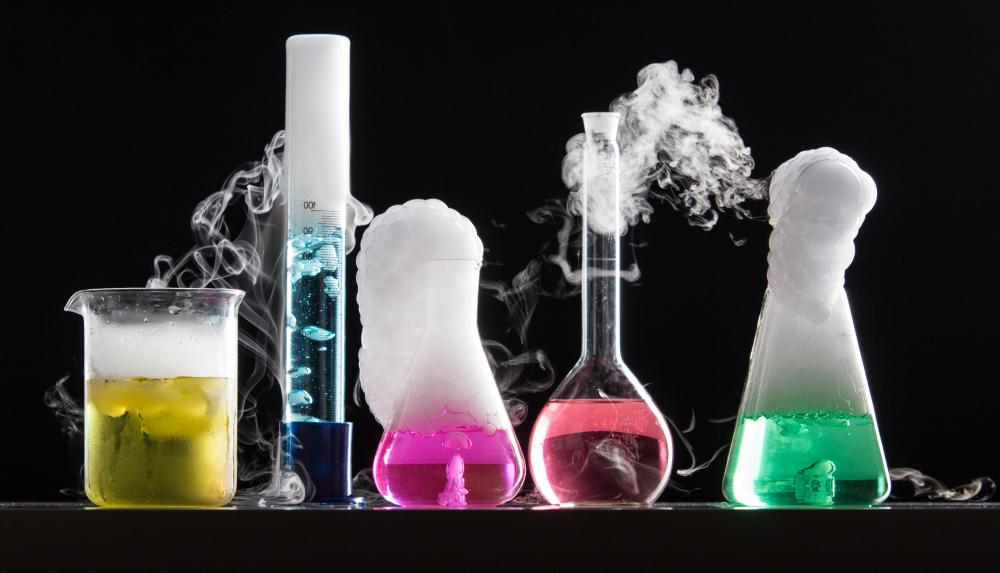# What is Kinetic Theory?

Niki Foster
Niki FosterAll gases are governed by kinetic theory to some extent but the theory does make some assumptions in order to function.

Kinetic theory is a scientific theory regarding the nature of gases. The theory goes by many names, including the kinetic theory of gases, kinetic-molecular theory, collision theory, and the kinetic-molecular theory of gases. It explains the observable and measurable, also called macroscopic, properties of gases in terms of their molecular composition and activity. While Isaac Newton theorized that the pressure of a gas is due to static repulsion between molecules, the kinetic theory holds that pressure is the result of collisions between molecules.Kinetic theory defines the pressure that is exerted by a gas in a container.

The kinetic theory makes a number of assumptions about gases. First, a gas is made of very small particles, each with non-zero mass, constantly moving in a random manner. The number of molecules in a gas sample must be large enough for statistical comparison.

Kinetic theory assumes that gas molecules are perfectly spherical and elastic, and that their collisions with the walls of their container are also elastic, meaning that they do not result in any change in velocity. The total volume of gas molecules is negligible as compared to the total volume of their container, meaning that there is ample space between the molecules. In addition, the time during the collision of a gas molecule with the container wall is negligible in relationship to the time between collisions with other molecules. The theory further relies on the assumption that any relativistic or quantum-mechanical effects are negligible, and that any effects of the gas particles on each other are negligible, with the exception of the force exerted by collisions. Temperature is the only factor affecting the average kinetic energy, or energy due to motion, of the gas particles.Collision theory, also known as kinetic theory, explains why certain chemical reactions take place.

These assumptions must be maintained in order for the equations of kinetic theory to function. A gas fulfilling all of these assumptions is a simplified theoretical entity known as an ideal gas. Real gases usually behave similarly enough to ideal gases for kinetic equations to be useful, but the model is not perfectly accurate.

Kinetic theory defines pressure as the force exerted by gas molecules as they collide with the container wall. Pressure is calculated as the force per area, or P = F/A. Force is the product of the number of gas molecules, N, the mass of each molecule, m, and the square of their average velocity, v2rms, all divided by three times the length of the container, 3l. Therefore, we have the following equation for force: F = Nmv2rms/3l. The abbreviation, rms, stands for root-mean-square, an average of the velocity of all the particles.

The equation for pressure is P = Nmv2rms/3Al. Since area multiplied by length is equal to volume, V, this equation can be simplified as P = Nmv2rms/3V. The product of pressure and volume, PV, is equal to two-thirds the total kinetic energy, or K, allowing the derivation of macroscopic properties from a microscopic one.

An important part of the kinetic theory is that kinetic energy varies in direct proportion to the absolute temperature of a gas. Kinetic energy is equal to the product of the absolute temperature, T, and the Boltzman constant, kB, multiplied by 3/2; K = 3TkB/2. Therefore, whenever temperature is increased, kinetic energy is increased, and no other factors have an effect on kinetic energy.Niki Foster

In addition to her role as a InfoBloom editor, Niki enjoys educating herself about interesting and unusual topics in order to get ideas for her own articles. She is a graduate of UCLA, where she majored in Linguistics and Anthropology.Niki Foster

In addition to her role as a InfoBloom editor, Niki enjoys educating herself about interesting and unusual topics in order to get ideas for her own articles. She is a graduate of UCLA, where she majored in Linguistics and Anthropology.

## You might also LikeProxy414

When certain gases collide, they create a greater amount of energy than other gases. That is why coals were mined for their great energy capacity when burnt. Coal mines all over the Western world were established to fuel the kinetic energy which drove production.

SilentBlue

What is matter? It seems that it is intrinsically related to spacetime. Only things which operate within spacetime can have any sort of force whatsoever, and can interact with energy. If gases are an aspect of matter, they are masses, which nevertheless can generate kinetic energy. The entire process of how energy and mass interact is almost on the edge of scientific understanding.

dbuckley212

Everything moves and exists in relation to other things around it. If there were nothing around a given atom, then there would be no way to tell if the atom were actually moving. The theory of general relativity states that all motion is relative. Energy is shown by things moving in relation to other things. In this process, the potential energy is released in the form of kinetic energy.

FitzMaurice

Using gases for power was the original basis of the steam engine. This revolutionized the way locomotives were powered and made the way for the steam engine train, which criss-crossed nations and powered industry. Using water and gas energy, people were able to power the engines of the future. Before this time, the only things that was truly powered by gas were smithy fires and church organs.